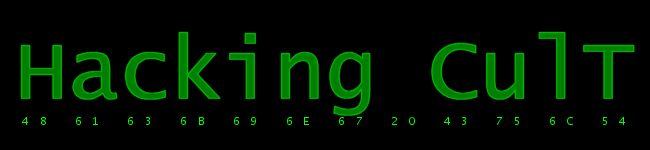Research / Megadrive Programming / Loops & arrays Loops in 68k are very limited... Forget about while- or for-like loops ;) For loops, you use DBcc. Usually, programmers just use DBF or DBRA (which mean the same). First operand is a data register that will be decremented and the second one is a label where to loop if this data register isn't -1. Example: ``` lea (\$1234).l,a0 lea (\$FFFF1234).w,a1 moveq #7,d0 Loop: move.w (a0)+,(a1)+ dbf d0,Loop``` This example will go back to the Loop label 8 times (d0 + 1). It's not that hard... I won't show the other DBcc instructions because they are practically useless. Maybe later, when I'll write an instruction list... Second part of this chapter will be arrays. Well, I call them arrays, but they really aren't. Those are just sets of bytes, words, longwords that are readed depending on some variable. For instance, we want to load a different music depending on the current level: ``` moveq #0,d0 ;clear d0 move.b (\$FFFFFE10).w,d0 ;move level to d0 (00 - GHZ, 01 - LZ etc) lea (MusicArray).l,a0 ;load the music values array into a0 move.b (a0,d0.w),d0 ;see the explanation rts MusicArray: dc.b \$81,\$82,\$83,\$84,\$85,\$86``` I thought 5 damn minutes about how should I explain this XD. The move.b (a0,d0.w),d0 instruction moves a byte at a0 + d0 to d0. Let's examine 3 possibilities (GHZ - 00, LZ - 01, MZ - 02) ```GHZ: d0 = 00 a0 = \$1234 move.b (a0,d0.w),d0 d0 = (a0 + d0) d0 = (\$1234 + 0) d0 = (\$1234) d0 = \$81 LZ: d0 = 01 a0 = \$1234 move.b (a0,d0.w),d0 d0 = (a0 + d0) d0 = (\$1234 + 01) d0 = (\$1235) d0 = \$82 MZ: d0 = 02 a0 = \$1234 move.b (a0,d0.w),d0 d0 = (a0 + d0) d0 = (\$1234 + 02) d0 = (\$1236) d0 = \$83``` I hope you get it... This chapter was short but very important, so if you don't understand something, read it 10 times =P And you can always ask on the forums. Back | Printer friendly<< 5. Editing the game code